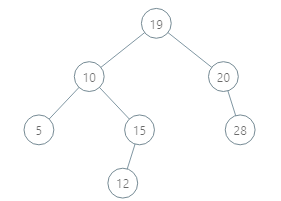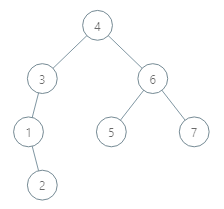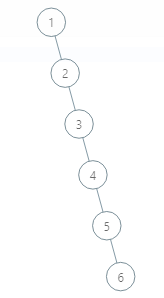10

# BST queries

Difficulty: EASYContributed By
Nishant Chitkara
Avg. time to solve
10 min
Success Rate
90%

Problem Statement
Suggest Edit

#### You are given an arbitrary binary search tree (BST) with N nodes numbered 1 to N, and each node is associated with a value. You are also given Q queries, each of the Q queries are of the form [ L, R ], your task is to find the number of nodes in the BST which lie in the range L to R for each query.

##### For example
``````You are given the following tree.
````````````And you are given 3 queries as follows
Q1 = [ 2 , 12 ]
Q2 = [ 10 , 50 ]
Q3 = [ 14 , 20 ]

Then the answers for them will be as follows.
Answer for Q1 = 3 i.e. nodes 5, 10 , 12.
Answer for Q2 = 6 i.e. nodes 10 , 12 , 15 , 19 , 20 , 28.
Answer for Q3 = 3 i.e. nodes 15 , 19 , 20.
``````
##### Note :
``````It is guaranteed that the tree given will be a binary search tree.
It is guaranteed that all the node values will be distinct.
``````
##### Input Format:
``````The first line of the input contains a single positive integer T, denoting the number of test cases.

The first line of each test case contains two positive integers N and Q, denoting the number of nodes in the BST and the number of queries respectively.

The second line contains the values of the nodes of the tree in the level order form ( 0 for NULL node) Refer to the example for further clarification.

The next Q lines of each test case contain two positive integers L and R as described in the problem statement.
``````

#### Example: Consider the binary tree``````The input of the tree depicted in the image above will be like:

4
3 6
1 0 5 7
0 2 0 0 0 0
0 0

Explanation :
7 is the number of nodes
The second line contains the value of nodes from 1 to 7.
Then the structure of the tree follows.
Level 1 :
The root node of the tree is 4

Level 2 :
Left child of 4 = 3
Right child of 4 = 6

Level 3 :
Left child of 3 = 1
Right child of 3 = null (0)
Left child of 6 = 5
Right child of 6 = 7

Level 4 :
Left child of 1 = null (0)
Right child of 1 = 2
Left child of 5 = null (0)
Right child of 5 = null (0)
Left child of 7 = null (0)
Right child of 7 = null (0)

Level 5 :
Left child of 2 = null (0)
Right child of 2 = null (0)

The first not-null node (of the previous level) is treated as the parent of the first two nodes of the current level. The second not-null node (of the previous level) is treated as the parent node for the next two nodes of the current level and so on.

The input ends when all nodes at the last level are null (0).
``````
##### Output Format:
``````For each query print a single integer on a new line, denoting the number of nodes in the tree with a value between L and R.
``````
##### Note:
``````You don't have to print anything, it has already been taken care of. Just Implement the given function.
``````
##### Constraints:
``````1 <= T <= 50
1 <= N, Q <= 3000
1 <= Value in a node <= 10^9
1  <= L <= R <= 10^9
Time Limit : 1 sec
``````
##### Sample Input 1:
``````1
6 3
1 0 2 0 3 0 4 0 5 0 6 0 0
3 4
3 8
1 6
``````
##### Sample Output 1:
``````2
4
6
``````
##### Explanation For Sample Input 1:
``````The above test case represents following bst
````````````And you are given 3 queries as follows
Q1 = [ 3 , 4 ]
Q2 = [ 3 , 8 ]
Q3 = [ 1 , 6 ]
Then the answers for them will be as follows.
Answer for Q1 = 2 i.e, nodes 3 , 4.
Answer for Q2 = 4 i.e, nodes 3 , 4 , 5 , 6.
Answer for Q3 = 6 i.e, nodes 1 , 2 , 3 , 4 , 5 , 6.
``````
##### Sample Input 2:
``````1
7 3
19 10 20 5 15 0 28 0 0 12 0 0 0 0 0
2 12
10 50
14 20
``````
##### Sample Output 2:
``````3
6
3
``````Console